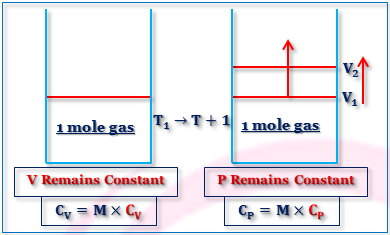## Heat Capacity Of Gases:

Heat capacity of a substance is defined as the amount of heat required to rise of the temperature by one degree. Heat capacity per gram of substance is called specific heat and per mole called molar heat capacity.
Thus, Molar heat capacity = Molar mass × Specific heat
CP = M × cp
CV = M × cv
Where CP and CV are the molar heat capacities at constant pressure and constant volume respectively. cp and cv are their specific heats.
The specific heat at constant pressure and constant volume are 0.125 and 0.075 Cal gm⁻¹K⁻¹ respectively. Calculate the molecular weight and atomicity of the gas. Name the gas if possible.
M = 40 and ⋎ = 1.66(mono-atomic), Ar(Argon).
For gases, there are two heat capacities at constant volume and constant pressure.
These are Represented as,
CV = (dq/dT)V = (dU/dT)V
CP = (dq/dT)P = (dU/dT)P + P(dV/dT)P
Difference in Heat Capacities of an Ideal Gas:
If the gas is assumed to be ideal, then,
PV = nRT and (dU/dT)V = (dU/dT)P
Again, P(dV/dT)P = nR
Thus for an ideal gas, PV = nRT; or, P(dV/dT)P = nR.
Again, CP = (dU/dT)P + P(dV/dT)P
or, CP = CV + {P×(nR/P)}
or, CP = CV +nR
For 1 mole ideal gas CP = Cv +R
Molar Heat capacities of Gases:Molar Heat Capacity Of Gases
From the above two descriptions, it is clear that CP and CV. Since for CP, some mechanical work is required as additional energy to absorbed for definite piston from volume V₁ to V₂. Thus , CP - CV = Mechanical Work = PdV = P(V₂-V₁) = PV₂-PV₁ = R(T+1) - RT = R
Again, CP = CV+R for 1 mole ideal gas.
Now let us find the expression of CV from the point of view of Kinetic Theory.
CV = Energy required to increase transnational kinetic energy of 1 mole gas for rise of 1° temperature + energy required to increase inter molecular energy of 1 mole gas for rise temperature of 1°.
Increase of transnational K.E. = (3/2)R(T+1) - (3/2)R = (3/2) R for 1 mole gas for 1° rise in temperature.
Mono-atomic Gases:
CV = (dU/dT)v = (3/2)R and CP = (5/2)R.
Thus γ = CP/CV = 5/3 ≃ 1.667
Poly-atomic Gases:
Linear  CV = (dU/dT)v = (3/2)R + R +(3N - 5)R
Non-linear  CV = (dU/dT)v = (3/2)R + (3/2)R +(3N - 6)R
Where N is the number of particles.
Molar Heat Capacities for Diatomic Molecules:
For diatomic molecule N = 2.
Thus CV = (3/2)R + R + R = (7/2)R and CP = (9/2)R.
∴ ⋎ = CP/CV = 9/7  ≃ 1.286
Molar Heat Capacities for Tri-atomic Molecules:
For tri-atomic molecule N = 3.
Thus CV(Linear) =